1800-212-7858 (Toll Free)
9:00am - 8:00pm IST all days
8104911739

or

Thanks, You will receive a call shortly.
Customer Support

You are very important to us

For any content/service related issues please contact on this toll free number

022-62211530

Mon to Sat - 11 AM to 8 PM

# R S Aggarwal and V Aggarwal Solution for Class 9 Mathematics Chapter 19 - Probability

Our RS Aggarwal & V Agarwal Textbook Solutions are considered extremely helpful for solving the tough questions which are asked in the CBSE Class 9 board exam. TopperLearning Textbook Solutions are compiled by subject experts. Herein, you can find all the answers to the textbook questions for Chapter 19 - Probability.

All our solutions are created in accordance with the latest CBSE syllabus, and they are amended from time to time to provide the most relevant answers. Our free RS Aggarwal & V Agarwal Textbook Solutions for CBSE Class 9 Mathematics will strengthen your fundamentals in this chapter and will help you in your attempts to score more marks in the final examination. CBSE Class 9 students can refer to our solutions any time — while doing their homework and while preparing for the exam.

Exercise/Page

## R S Aggarwal and V Aggarwal Solution for Class 9 Mathematics Chapter 19 - Probability Page/Excercise MCQ

Solution 1

Correct option: (d)

Total number of people = 645

Number of people having high school certificate = 516Solution 2

Correct option: (c)

Total number of students = 11 + 15 + 8 + 6 = 40

Number of students having blood group AB = 8Solution 3

Correct option: (d)

Total number of bulbs = 80

Number of bulbs having life of 1150 hours = 0

Required probability = 0

Solution 4

Correct option: (c)

Total number of children = 364

Number of children who like to eat potato chips = 91

Number of children who do not like to eat potato chips = 364 - 91 = 273Solution 5

Correct option: (b)

Total number of outcomes = 1000Solution 6

Correct option: (b)

Total number of bulbs = 80Solution 7

Correct option: (c)

Total number of students = 200

Number of students who does not like Sanskrit = 65Solution 8Solution 9Solution 10Solution 11Solution 12Solution 13Solution 14Solution 15Solution 16

Correct option: (c)

Total number of outcomes = 600## R S Aggarwal and V Aggarwal Solution for Class 9 Mathematics Chapter 19 - Probability Page/Excercise 19

Solution 1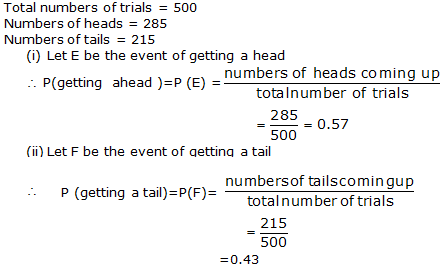Solution 2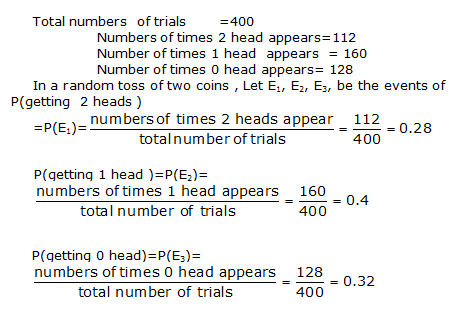Solution 3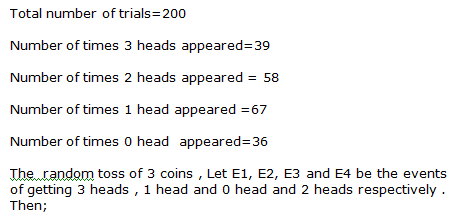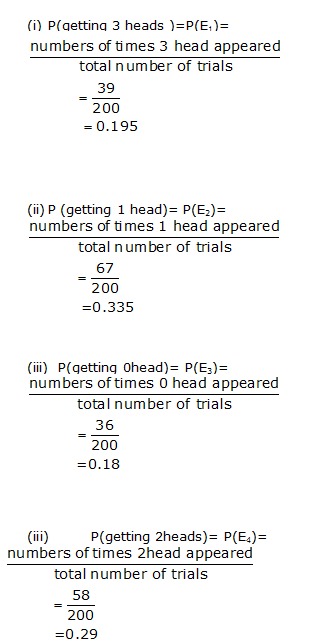Solution 4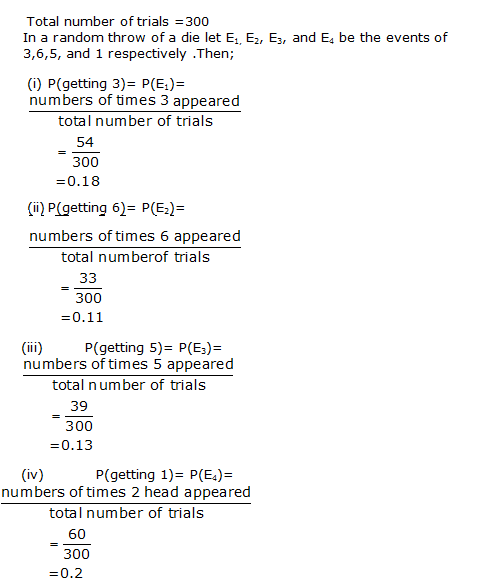Solution 5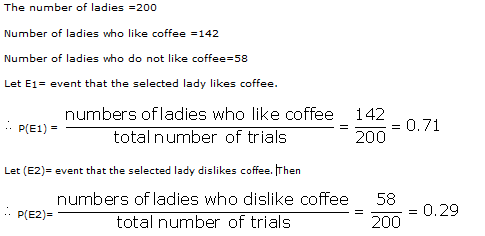Solution 6

Number of tests in which he gets more than60% marks =2

Total numbers of tests =6Required probabilitySolution 7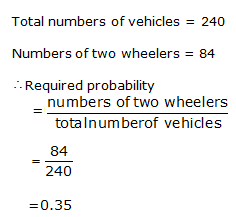Solution 8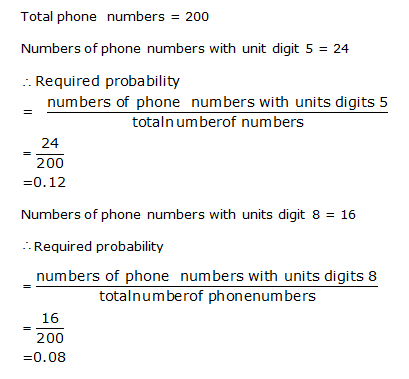Solution 9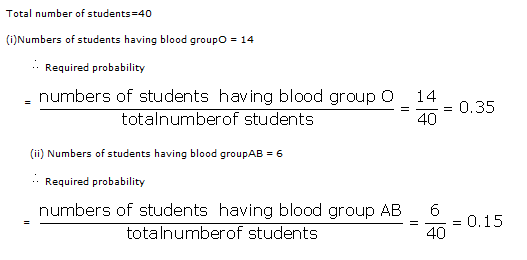Solution 10

Total number of salt packets = 12

Number of packets containing more than 2 kg of salt = 5

Therefore,

Probability that the chosen packet contains more than 2 kg of saltSolution 11

Total number of ball played = 30

Number of times boundary was hit = 6

Number of times boundary was not hit = 30 - 6 = 24

Therefore,

Probability that the batsman did not hit the boundarySolution 12Solution 13Solution 14Solution 15Solution 16Solution 17

Total number of electric bulbs = 800

Number of defective bulbs = 36

Number of non-defective bulbs = 800 - 36 = 764

Hence, probability that the bulb chosen is non-defectiveSolution 18

Fill in the blanks.

(i) Probability of an impossible event = 0

(ii) Probability of a sure event = 1

(iii) Let E be an event. Then, P(not E) = 1 - P(E)

(iv) P(E) + P(not E) = 1

(v) 0 ≤ P(E) ≤ 1

## Browse Study Material

### Browse questions & answers

TopperLearning provides step-by-step solutions for each question in each chapter in the RS Aggarwal & V Aggarwal Textbook. Access the CBSE Class 9 Mathematics Chapter 19 - Probability for free. The questions have been solved by our subject matter experts to help you understand how to answer them. Our RS Aggarwal Solutions for class 9 will help you to study and revise the whole chapter, and you can easily clear your fundamentals in Chapter 19 - Probability now.

# Text Book Solutions

CBSE IX - Mathematics

## This content is available for subscribed users only.

OR

Call us

1800-212-7858 (Toll Free) to speak to our academic expert.
OR

Let us get in touch with you